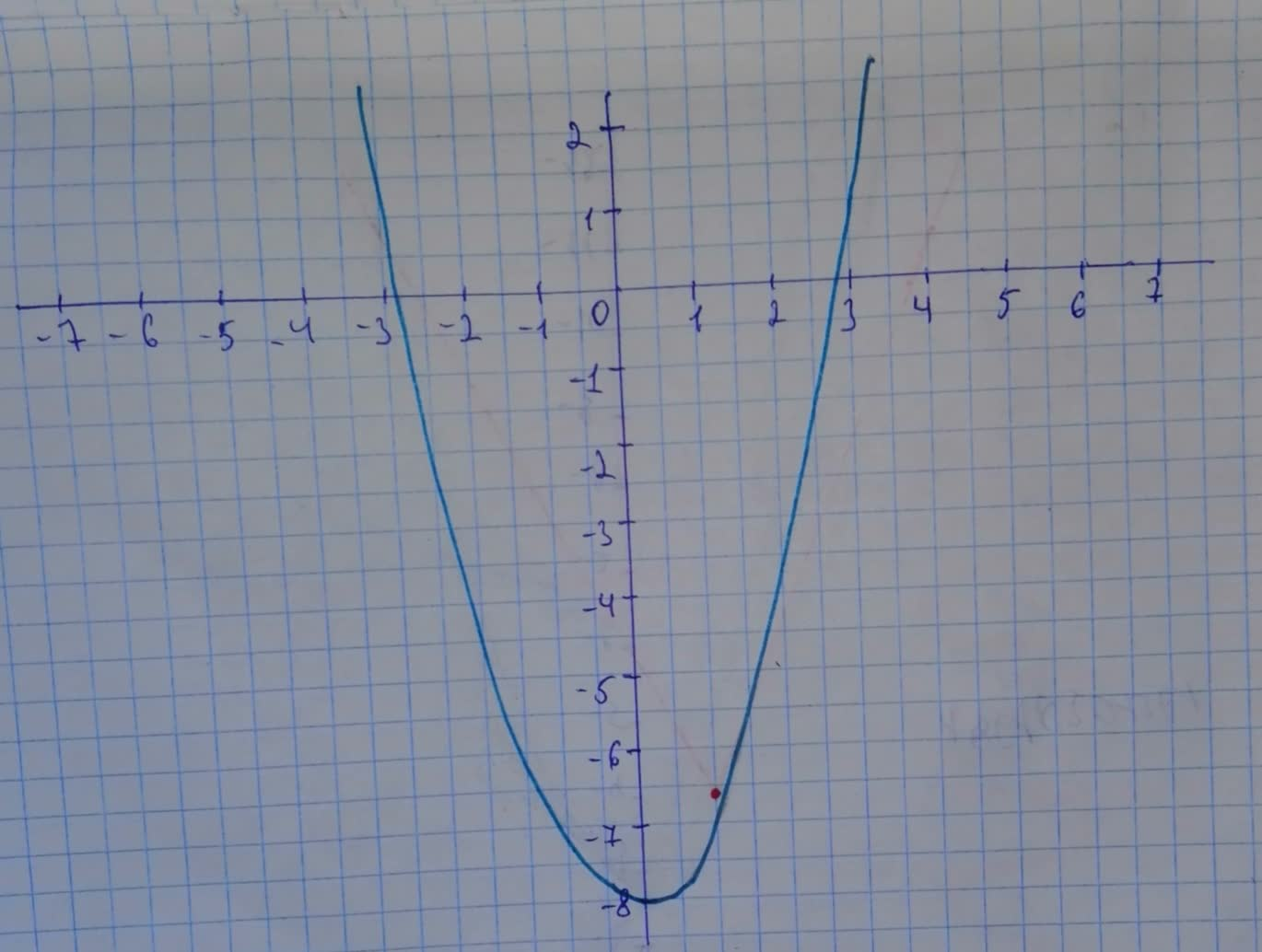# h is related to one of the six parent functions. a) Identify the parent function f. b) Describe the sequence of transformations from f to h. c) Sketch the graph of h by hand. d) Use function notation to write h in terms of the parent function f. h(x)=(-x)^{2}-8boitshupoO 2021-01-27 Answered
h is related to one of the six parent functions.
a) Identify the parent function f.
b) Describe the sequence of transformations from f to h.
c) Sketch the graph of h by hand.
d) Use function notation to write h in terms of the parent function f.
$h\left(x\right)={\left(-x\right)}^{2}-8$
You can still ask an expert for help

• Questions are typically answered in as fast as 30 minutes

Solve your problem for the price of one coffee

• Math expert for every subject
• Pay only if we can solve itlamusesamuset
a) Parent function: $f\left(x\right)={x}^{2}$
b) Reflection in the y-axis
Vertical shift 8 units downward
c) The graph will bed) In function,